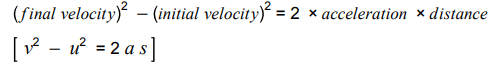# AQA GCSE Physics Acceleration

## Resources

The specification will tell you most of the answers to these questions. The fun starts on page 50 – https://filestore.aqa.org.uk/resources/physics/specifications/AQA-8463-SP-2016.PDF

2. You may also find the BBC Bitesize website helpful also. https://www.bbc.co.uk/bitesize/guides/zwc7pbk/revision/1

## Questions

1. Write down an equation to calculate the average acceleration of a moving object?
2. What are the units of acceleration?
3. What is meant by a change in velocity?
4. What word can be used to describe an object that is slowing down?
5. Research some typical accelerations using the units given in Q2. For instance, an aeroplane taking off or a train decelerating
6. What does the gradient of a velocity-time graph tell us?
7. What does the area underneath a velocity-time graph tell us? The area is often a triangle.
8. The area underneath a graph can be sometimes calculated using a method of counting squares. What is meant by this?
9. Do you know this equation?10. What are units of velocity? What are the units of distance? What are the units of acceleration?
11. What is the value of gravity near the Earth’s surface for any object falling freely under gravity?
12. An object falling through a fluid initially accelerates due to the force of gravity. Eventually, the resultant force will be zero and the object will move at its terminal velocity. Sketch a graph of velocity against time for a skydiver. Label all the important points and explain what is happening at each point in terms of forces. Air resistance and weight are important forces to consider.